output.to from Sideway
Finite Element

1D Element in 2D Frame

Draft for Information Only

# Content

` Finite Element Analysis   One Dimensional Linear Element: in 2D Frame   Element   Shape Function or Interpolation Function  The Material Law or Constitutive Equations  The Element Stiffness Matrix   Force Equilibrium Approach   Minimum Total Potential Energy Approach  One Dimensional Linear Element: Example in 2D Frame   Element-wise Force-Displacement Equation   Element 1   Element 2   Element 3  Node-Wise Force-Displacement Equation    node 1   node 2   node 3  Global Force-Displacement Equation  Boundary Conditions and External Loads  Global Conditionalized Force-Displacement Equation  Force-Displacement Equation Solving `

## Finite Element Analysis

One dimensional linear element can be used to analysis structural pin jointed problems.

### One Dimensional Linear Element: in 2D Frame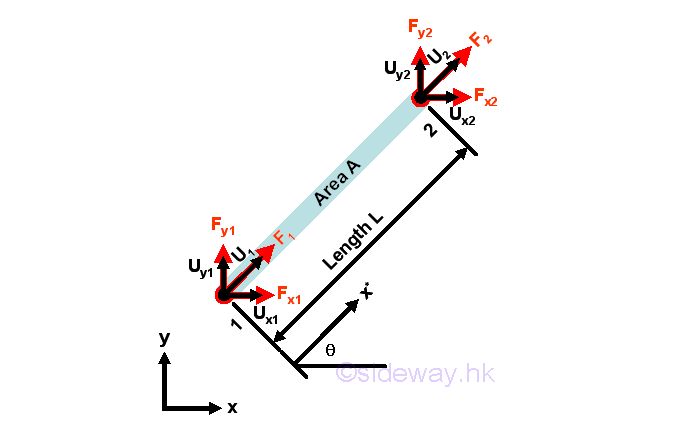### Element

In an 2D environment, a typical bar element of length L and cross-sectional area A is assumed to be oriented at an angle θ anticlockwise from x-axis. The forces acting upon the bar element, which can be expressed as an element vector, can be decomposited such that forces between each element can react to each other.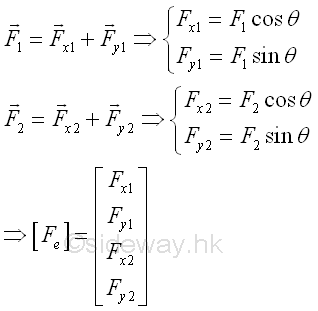The displacements of the nodes of the bar element, which can be expressed as an element vector, can be decomposited and expressed in terms of decomposited elements such that displacements due to the bar element can be connected with other elements.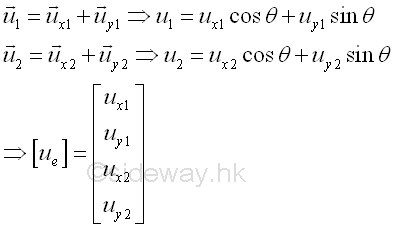### Shape Function or Interpolation Function

For a two-node linear element, the linear displacement function can be assumed to be a linear function of x* along the axial direction of the element  That is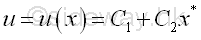Similar to other boundary problems, this trial displacement function for the bar must satisfy the boundary displacement conditions at two nodes. That is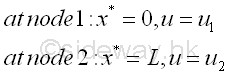The displacement is therefore equal to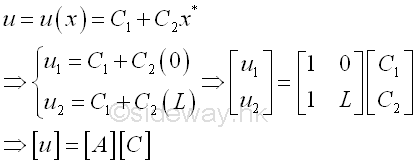where [u] is the displacement vector of the element, [A] is a 'coordinate matrix', and [C] is the constant vector.

The constant matrix is therefore equal to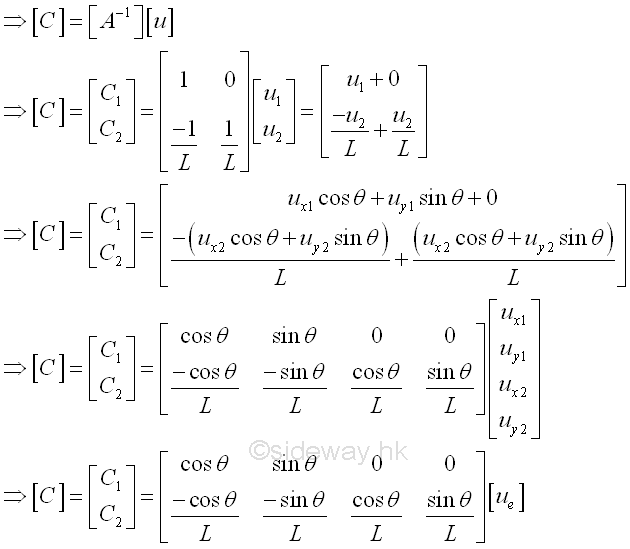The displacement function and shape function are therefore equal to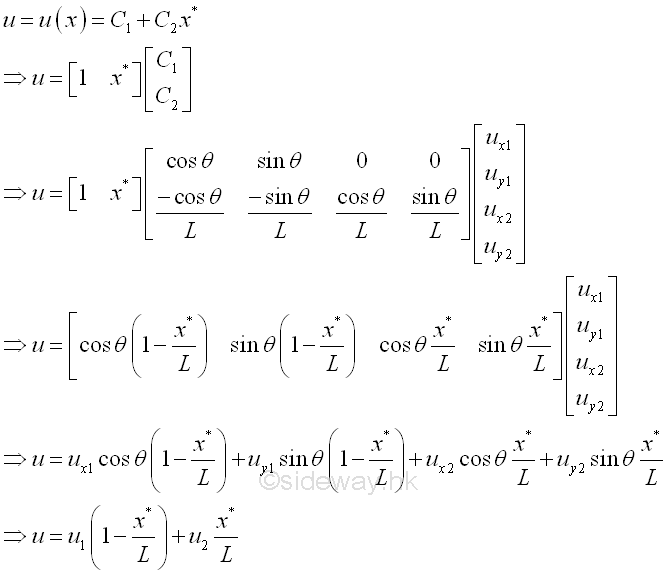### The Material Law or Constitutive Equations

Once the choice of shape functions is fixed, the type of the finite element is also determined. The finite element should also follow the law of material, that is the stress-strain relationships.

The strain in a bar element is given by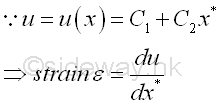The strain of the element is therefore equal to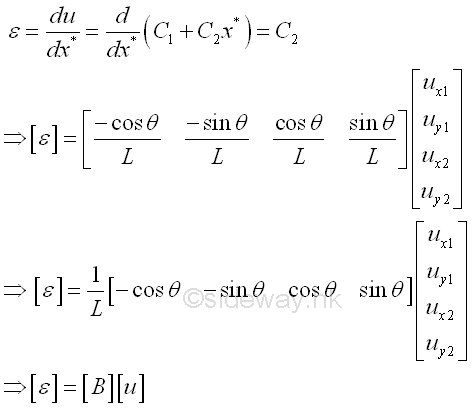Where [B] is the strain displacement dimension matrix.

Since the bar element is under uniaxial load, the stress in a bar element is given by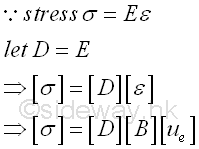Where [D] is the material property or stress-strain matrix and is equal to the Young's modulus E for a uniaxial load. The stress is then expressed in terms of displacement also.

### The Element Stiffness Matrix

#### Force Equilibrium Approach

Since the element is in equilibrium, the resultant force acting upon the element should be equal to zero. or forces act on the element is equal and opposite. Imply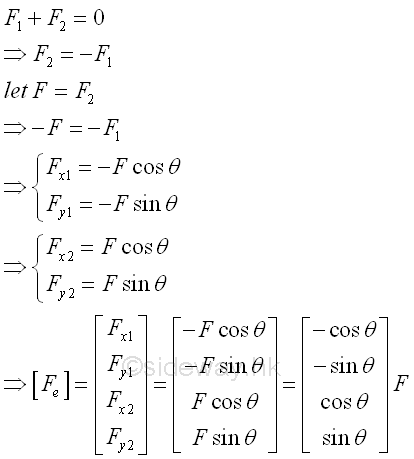The element force vector can also be expressed in terms of the strain displacement dimension matrix [B], imply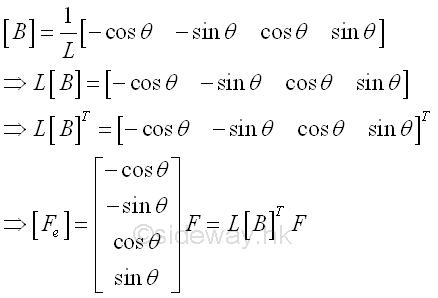Since the element is under an uniaxial load F, the stress in the element is equal to,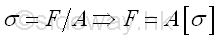Therefore the element force vector can further be expressed in terms of displacement,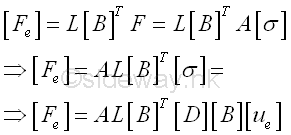Therefore the element force vector can be expressed in form of stiffness times displacement,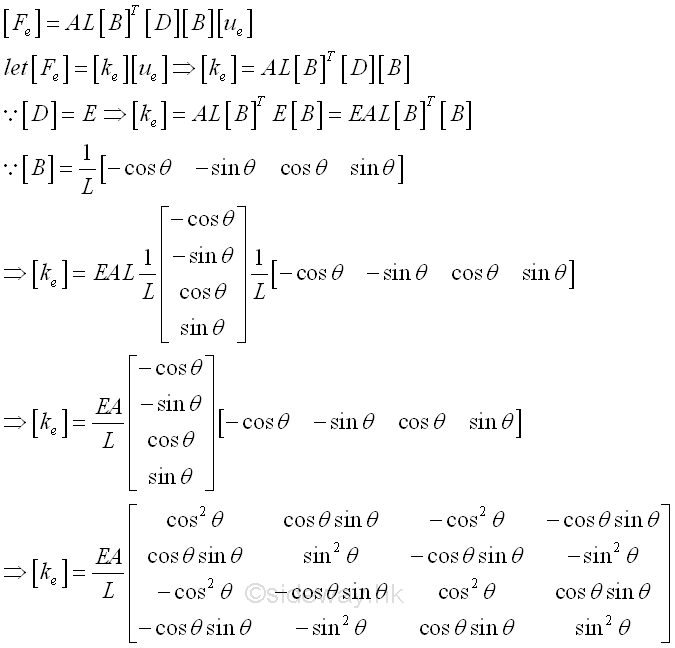and the element stiffness matrix is a symmetric matrix.

#### Minimum Total Potential Energy Approach

By neglecting the body weight of the bar, the total potential enegy of the element is,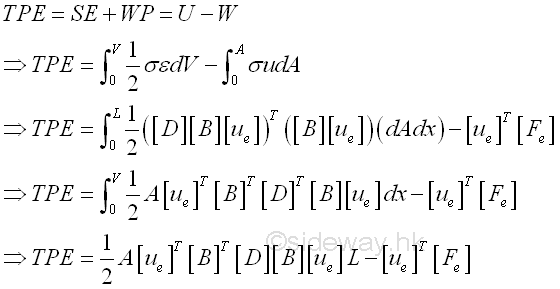By the principle of minimum total potential energy, the differential of total potential energy (TPE) with respect to the displacement [Ue] must be zero, imply,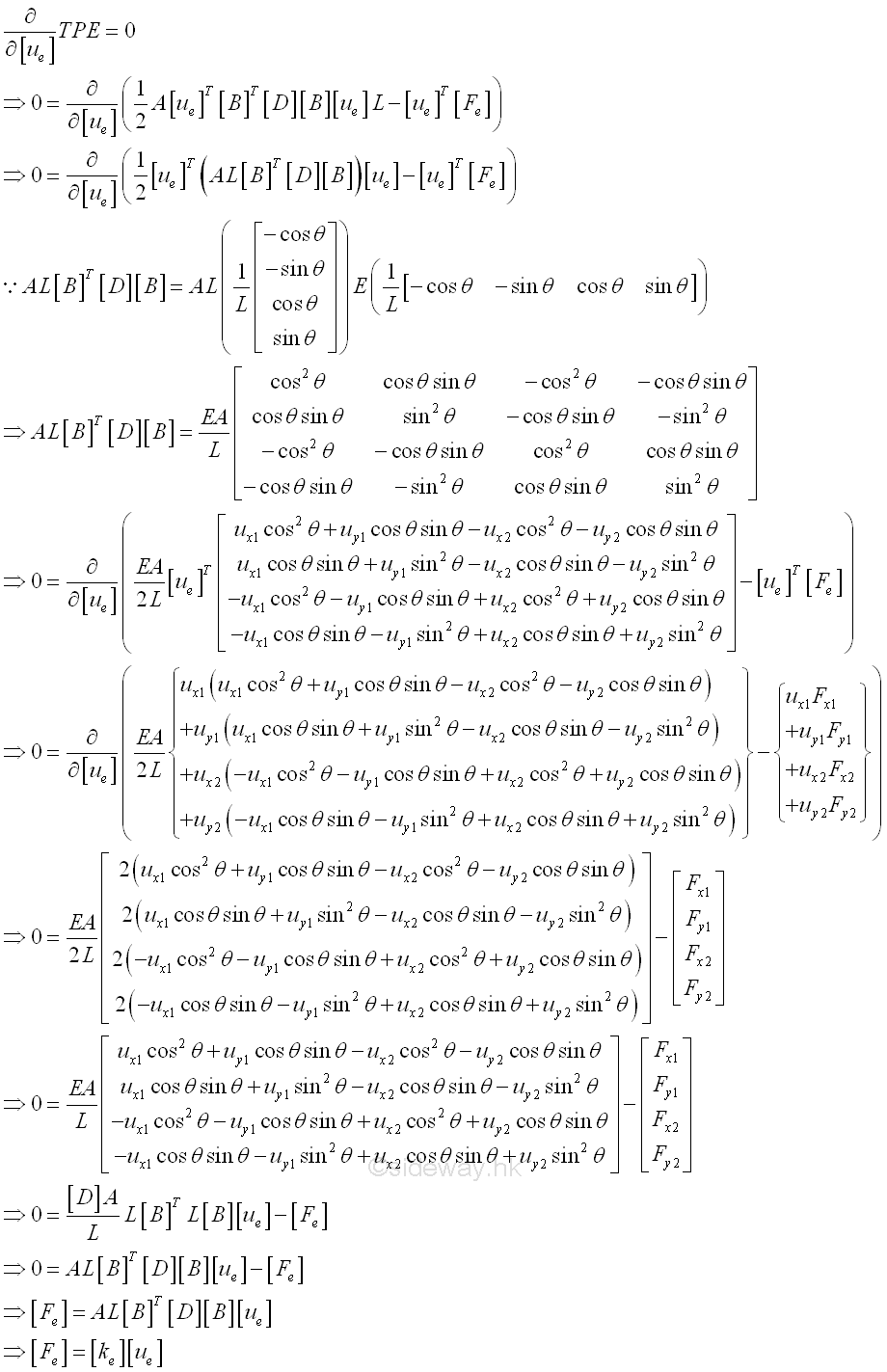### One Dimensional Linear Element: Example in 2D Frame

Consider a simple system with 3 elements of same material of Young's modulus E, length L and area A. Three elements are connected by hinges. Three elements are arranged in an equilateral triangle shape, with one end of element 1 is fixed to a wall and the other end of element 1 is supported by a roller, while there is an applied load F applied to the top hinge of the triangular structure.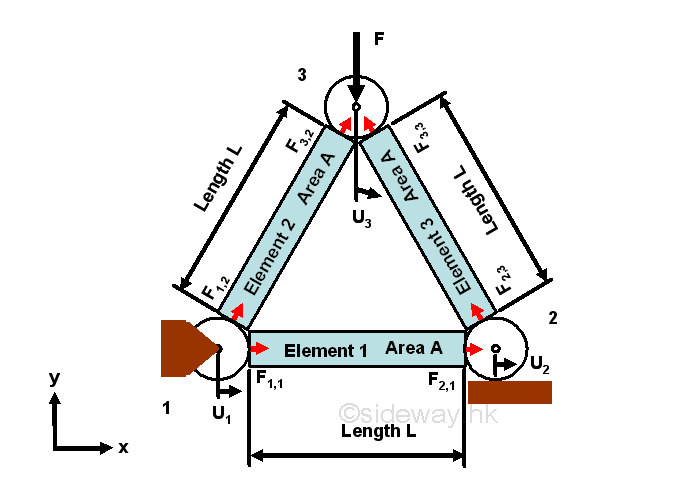### Element-wise Force-Displacement Equation

Element-wise Force-Displacement Equations for each element of the system can be constructed accordingly. Besides the length, cross-sectional area, and material property of each element, the orientation of the element is another important property of the element. For consistency, positive angle are used to orient the element at an angle θ anticlockwise from x-axis.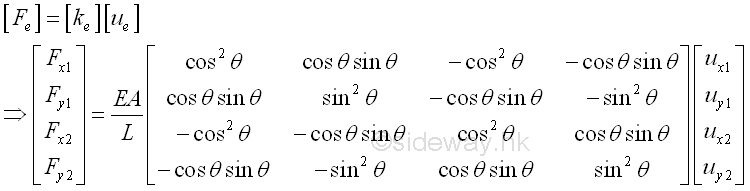#### Element 1

For element 1, that is from node 1 to node 2, angle of orientation is 0 degree, length=L, area=A, Young's modulus=E, imply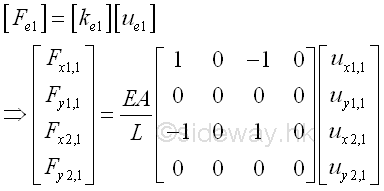#### Element 2

For element 2, that is from node 1 to node 3, angle of orientation is 60 degree, length=L, area=A, Young's modulus=E, imply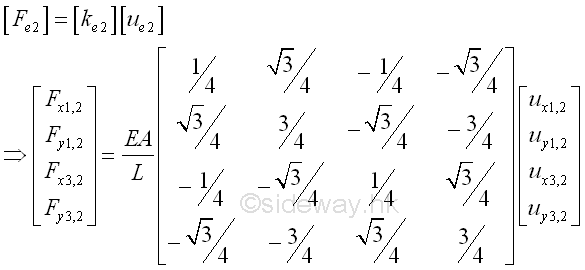#### Element 3

For element 3, that is from node 2 to node 3, angle of orientation is 120 degree, length=L, area=A, Young's modulus=E, imply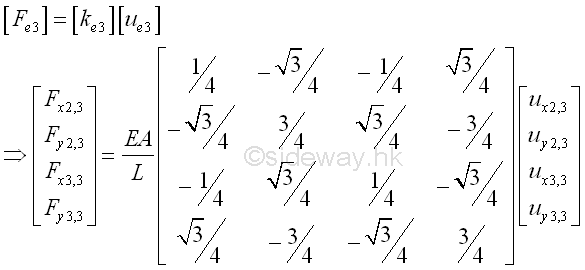### Node-Wise Force-Displacement Equation

For both the force equilibrium approach or the minimum potential energy approach, all element-wise force-displacement equations are in equilibrium. Since the displacement of any node in the system is same for every element attached to the node, the element-wise displacements of nodes are the same and with common displacement for every element connected. However, the considered element-wise force is not common force for every element connected to the node, and the forces for each element, in general, are usually difference in magnitude and direction. All element forces at a particular node are used to create the corresponding work potential for all element connected to the node, therefore the sum of all element forces are equal to induced forces due to the displacements of all elements connect to the node.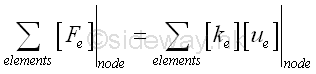#### node 1

Two elements, 1 and 2, are connected at node 1. The sum of element forces at node 1 is equal to the sum of induced forces due to the displacements of all elements, 1 and 2, connect to the node 1.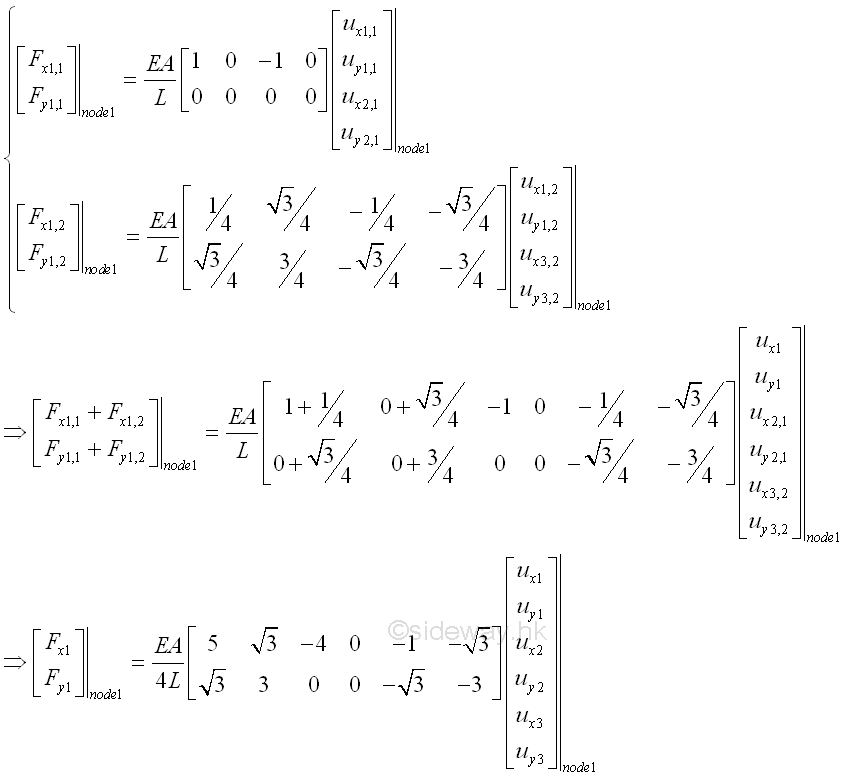#### node 2

Two elements, 1 and 3, are connected at node 2. The sum of element forces at node 2 is equal to the sum of induced forces due to the displacements of all elements, 1 and 3, connect to the node 2.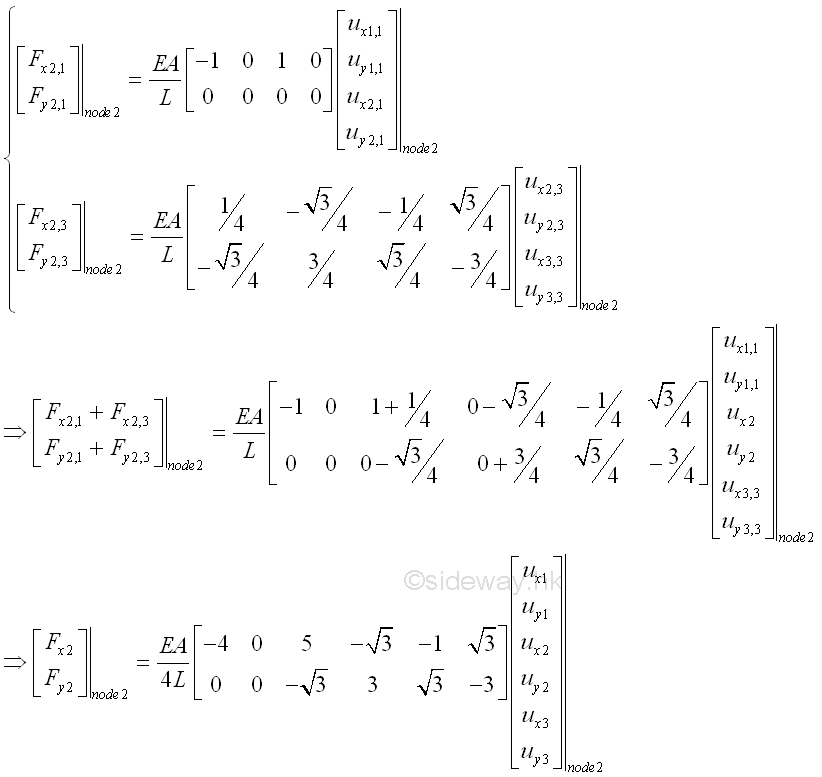#### node 3

Two elements, 2 and 3, are connected at node 3. The sum of element forces at node 3 is equal to the sum of induced forces due to the displacements of all elements, 2 and 3, connect to the node 3.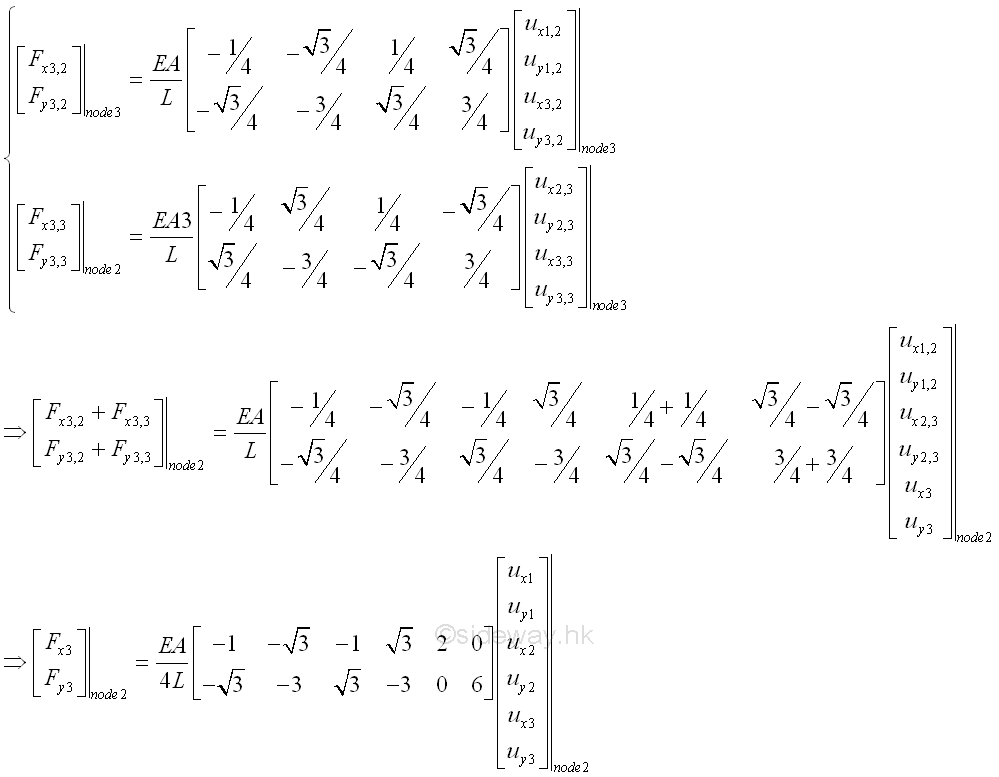### Global Force-Displacement Equation

All element forces can be assembled as a global system of equations in form of global matrices which contains all the nodes.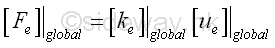That is 2*3 element forces, 2*3 displacements at 3 nodes, and stiffness matrix is (2*3) by (2*3). imply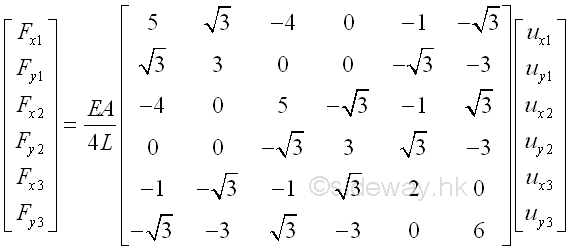and the global stiffness matrix is a symmetric matrix.

### Boundary Conditions and External Loads

Since the system of elements is in equilibrium, both forces and displacement at node should be in equilibrium. In other words, The displacements of nodes in the global force-displacement equations should be same as the allowable boundary conditions, and the element forces exerted on nodes should be equal to the external load acted upon the nodes accordingly. That is,

•  Fixed hinge at node 1. Fixed or no displacement on both x and y axes are allowed.

Imply ux1=uy1=0

• Horizontal sliding hinge at node 2. No download movement or no displacement on y axis is allowed at node 2 in this case.

Imply uy2=0

• Free or displacements on both x and y axes are allowed at node 3.

• Only reactions due to fixed hinge at node1.

Imply Fx1=Rx1; Fy1=Ry1.

• Only vertical reaction along y axis due to horizontal sliding hinge at node 2.

Imply Fx2=0; Fy2=Ry2.

• Only external downward force F and no reaction due to free at node 3.

Imply Fx3=0; Fy3=-F.

### Global Conditionalized Force-Displacement Equation

Substitute all boundary conditions and external loads into the global force-displacement equation. That is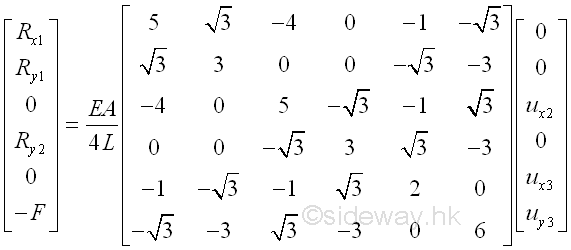### Force-Displacement Equation Solving

There are 6 force-displacement equations and 6 unknowns, therefore the global force-displacement equation can be solved. Since the most interested unknown variables are displacements, the global force-displacement equation can be divided into two parts. Force-displacement equations with unknown displacement variables and force-displacement equations with unknown reaction variables.

For force-displacement equations with unknown reaction variables which also depending on unknown displacement variables.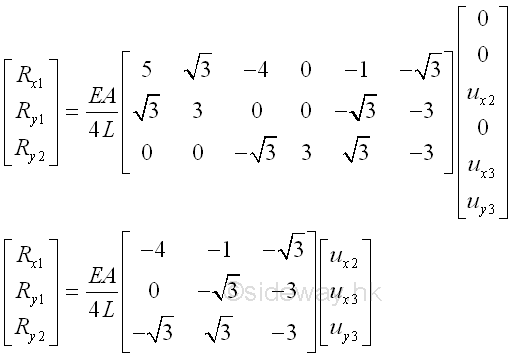For force-displacement equations with unknown displacement variables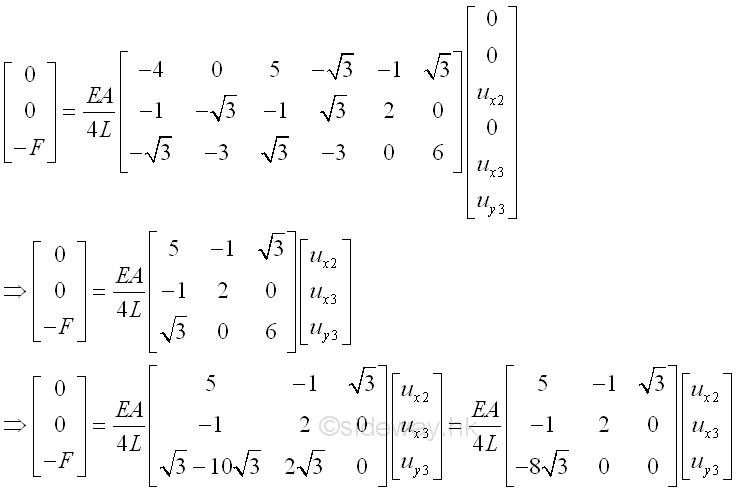Unknown displacement variables are therefore equal to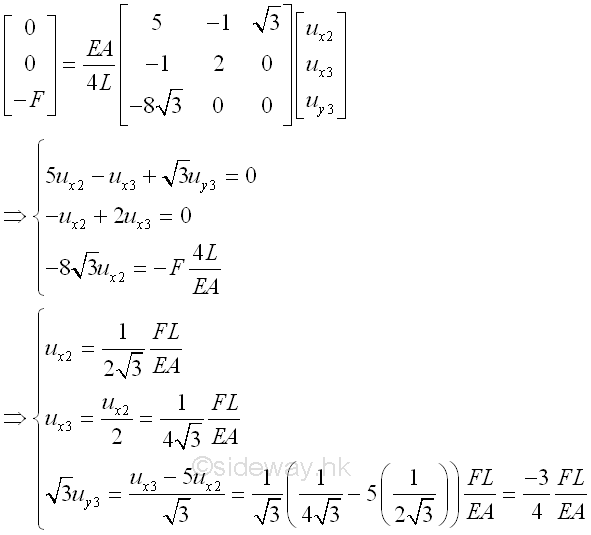Unknown reaction variables are therefore equal to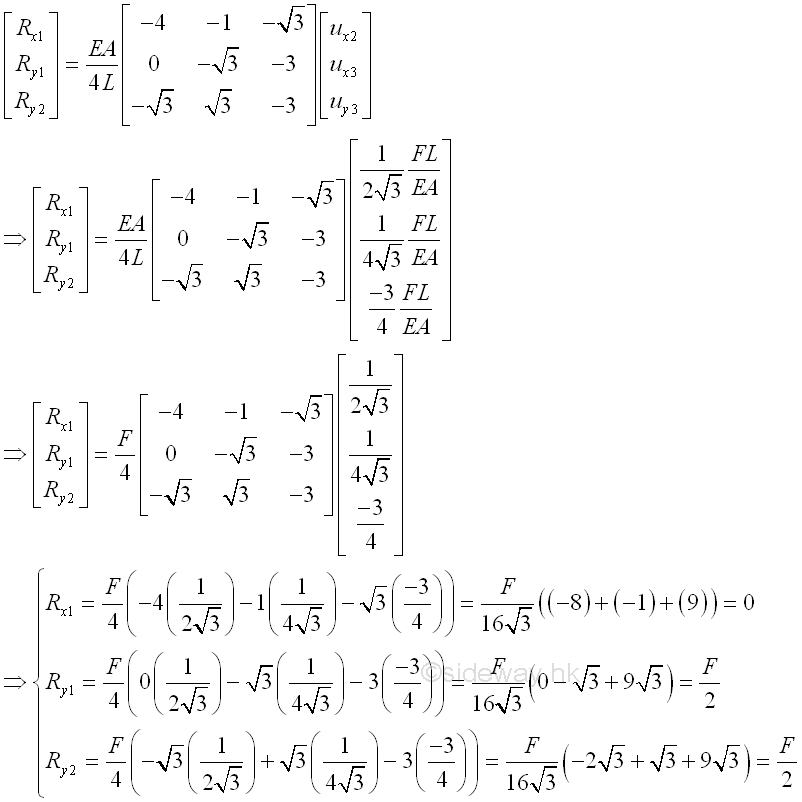©sidewayReferences

1. Paulo Ribenboim, 2000, My Numbers, My Friends: Popular Lectures on Number Theory, Springer-Verlag, New York
2. Kenneth H. Rosen, 2012, Discrete Mathematics and Its_Applications, McGraw-Hill, New YorkID: 140600017 Last Updated: 2014/7/4 Revision: 1 Ref:Home (5)

Business

Management

HBR (3)

Information

Recreation

Hobbies (7)

Culture

Chinese (1097)

English (336)

Reference (66)

Computer

Hardware (149)

Software

Application (187)

Digitization (24)

Numeric (19)

Programming

Web (644)CSS (SC)

ASP.NET (SC)

HTML

Knowledge Base

Common Color (SC)

Html 401 Special (SC)

OS (389)MS Windows

Windows10 (SC)

.NET Framework (SC)

DeskTop (7)

Knowledge

Mathematics

Formulas (8)

Number Theory (206)

Algebra (20)

Trigonometry (18)

Geometry (18)

Calculus (67)

Complex Analysis (21)

Engineering

Tables (8)

Mechanical

Mechanics (1)

Rigid Bodies

Statics (92)

Dynamics (37)

Fluid (5)

Control

Acoustics (19)

FiniteElement (2)

Biology (1)

Geography (1)

Latest Updated Links

Copyright © 2000-2019 Sideway . All rights reserved Disclaimers last modified on 10 Feb 2019